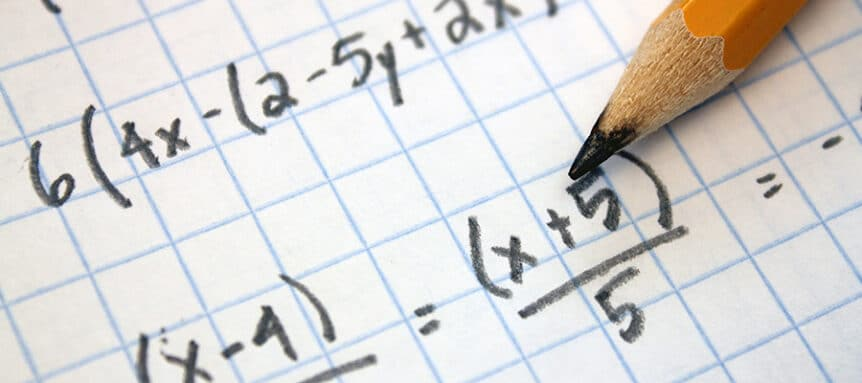# Basic Mathematics

Tanya Haun

Current Status
Not Enrolled
Price
\$\$70
Get Started

### Course Description:

Solve mathematical problems requiring simple addition, subtraction, multiplication, and division. You will also be able to round to the correct number of significant figures, calculate percentages, read graphs, and use simple geometric definitions and formulas.

2 Hours Continuing Education

This course requires that a minimum total time of 110 minutes is spent in the coursework. When you are in a lesson, you must spend the required time in the lesson before you will be permitted to proceed to the next page. If you need to take a break, you can do so in between each lesson.

The lessons are set to a minimum time but you can take as much time as you need beyond that. Don’t rush through the material in the lessons and feel free to take notes.